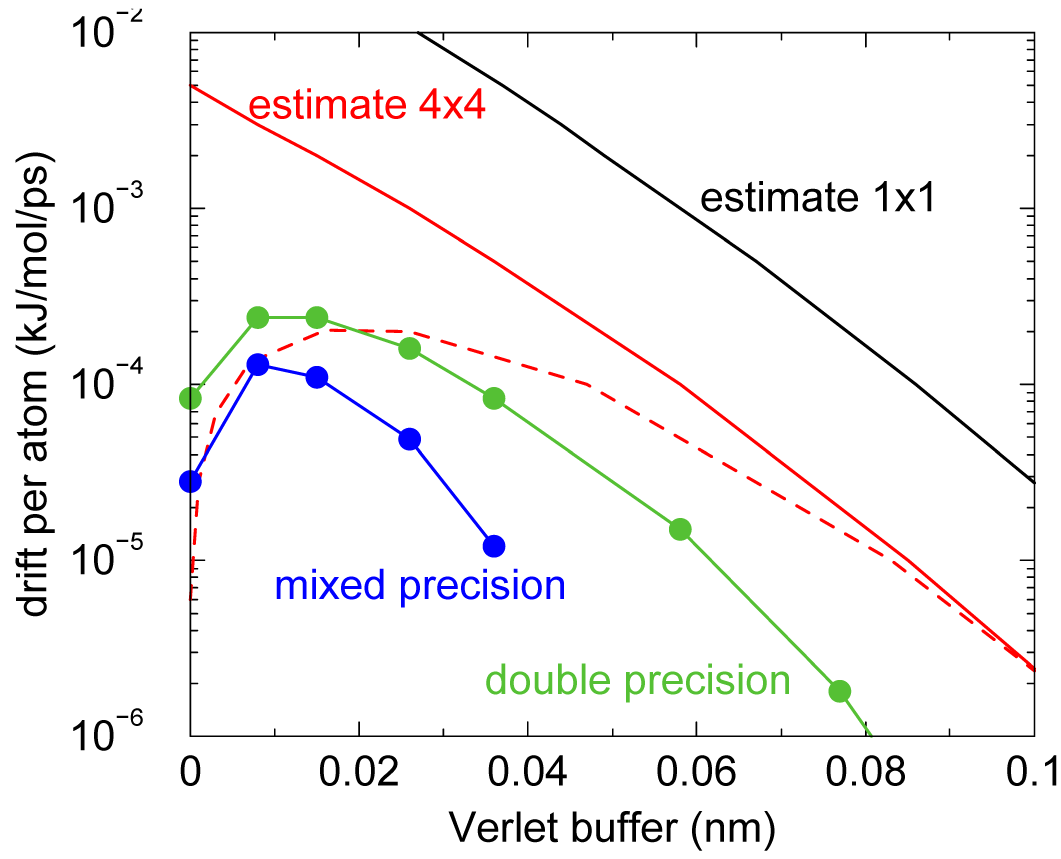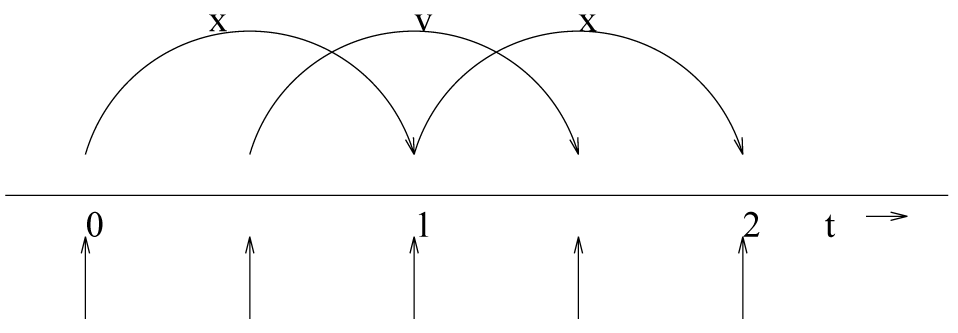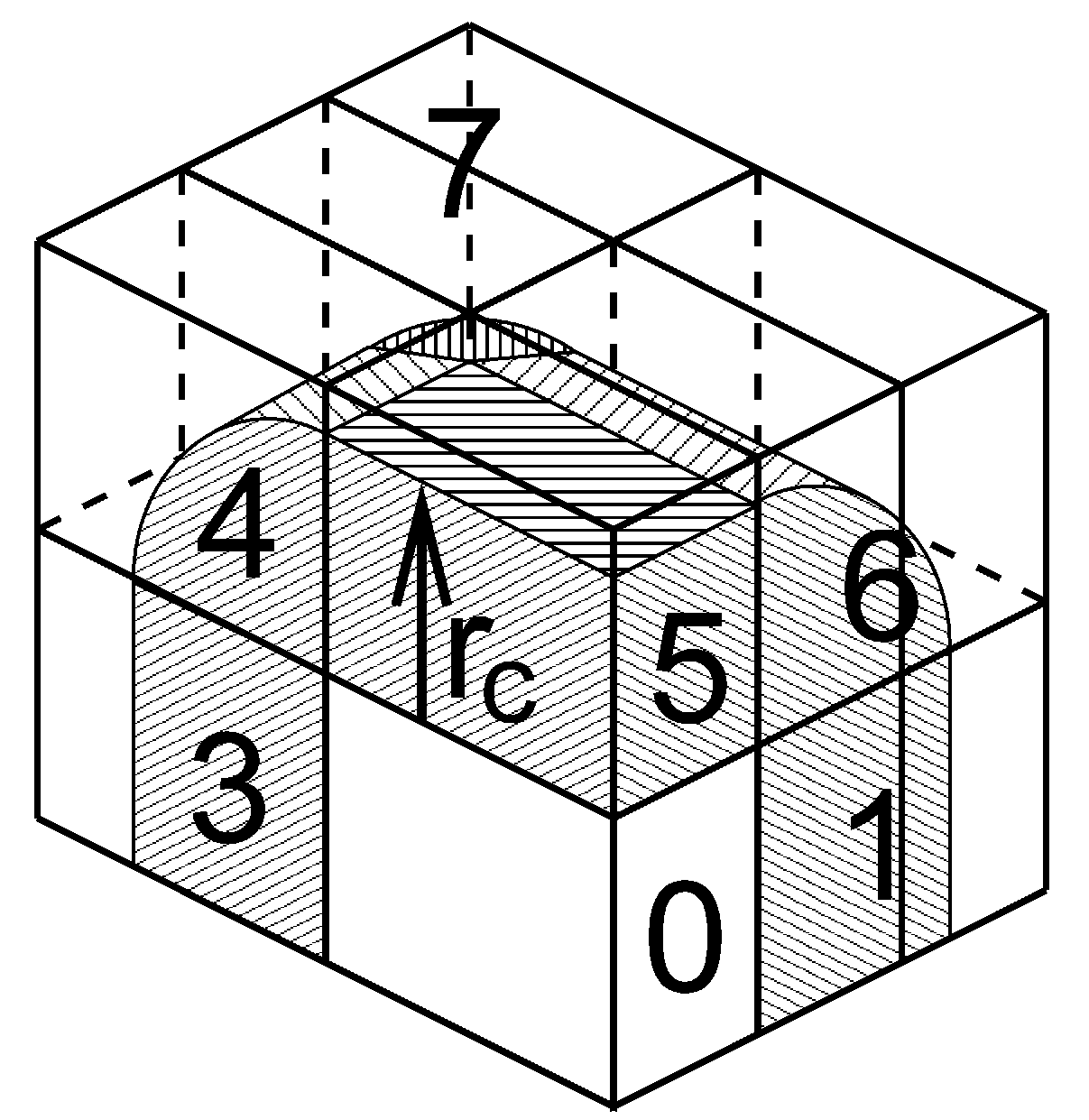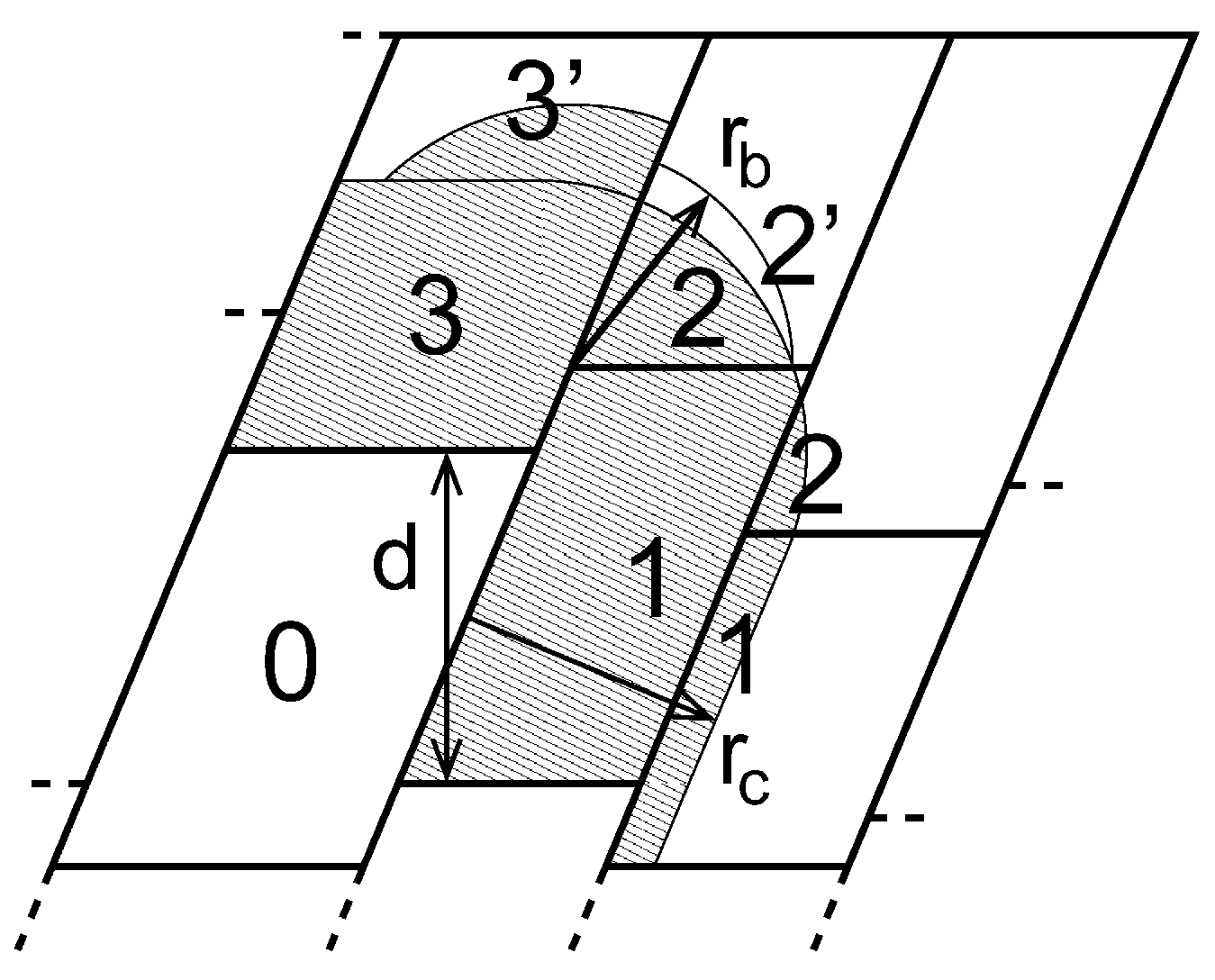# GROMACS中文手册：第三章　算法

## 3.2 周期性边界条件

GROMACS使用周期性边界条件, 并结合了 最小映像约定: 对短程非键相互作用, 每个粒子只考虑它的一个最近的映像. 对长程静电相互作用, 这有时不够准确, 因此GROMACS还采用了点阵加和的方法, 如Ewald加和, PME和PPPM.

GROMACS支持任何形状的三斜盒子. 模拟盒(元胞)由3个盒矢量 $$\mathbf{a,b,c}$$ 定义. 盒矢量必须满足以下条件:

$a_y=a_z=b_z=0 \tag{3.1}$

$a_x > 0, \; b_y > 0, \; c_z > 0 \tag{3.2}$

$\abs{b_x} \le {1\over2} a_x, \; |c_x| \le {1\over2} a_x, \; |c_y| \le {1\over2} b_y \tag{3.3}$

0 $$d$$ 0
0 0 $$d$$
$$90^{\circ}$$ $$90^{\circ}$$ $$90^{\circ}$$

$$0.707d^3$$
$$d$$ 0 $${1\over2}d$$
0 $$d$$ $${1\over2}d$$
0 0 $${1\over2}\sqrt2 d$$
$$60^{\circ}$$ $$60^{\circ}$$ $$90^{\circ}$$

0 $${1\over2}\sqrt3 d$$ $${1\over6}\sqrt3 d$$
0 0 $${1\over3}\sqrt6 d$$

$$0.770 d^3$$
$$d$$ $${1\over3}d$$ -$${1\over3}d$$
0 $${2\over3}\sqrt2 d$$ $${1\over3}\sqrt2 d$$
0 0 $${1\over3}\sqrt6 d$$
$$71.53^{\circ}$$ $$109.47^{\circ}$$ $$71.53^{\circ}$$

### 3.2.2 截断限制

$R_c < {1\over2} \text{min} (\lVert \mathbf a \rVert, \lVert \mathbf b\rVert, \lVert \mathbf c\rVert) \tag{3.4}$

$R_c < \text{min}(a_x, b_y, c_z) \tag{3.5}$

$R_c < {1\over2} \text{min}(a_x, b_y, c_z) \tag{3.6}$

## 3.3 组的概念

GROMACS的MD和分析程序可对用户自定义的原子 进行一些操作. 组的最大数目为256, 但每个原子最多只能属于六类不同的组. 这六类组如下:

GROMACS工具中组的使用将在8.1节详述.

## 3.4 分子动力学

MD的整个流程见图3.3. 每次运行MD或EM都需要体系中所有粒子一组初始坐标和(可选的)初始速度作为输入. 本章不介绍如何获得它们; 对于如何设置并实际运行MD, 请参考www.gromacs.org上的在线手册.

### 3.4.1 初始条件

$p(v_i)=\sqrt{ {m_i \over 2\p kT } } \exp\left(-{m_iv_i^2 \over 2kT} \right) \tag{3.7}$

### 3.4.2 近邻搜索

• 原子在两次近邻搜索之间穿过了截断区域, 和/或
• 对包含一个以上原子的电荷组, 当电荷组的几何中心移动到截断距离以外/内时, 会导致一部分原子对移动到截断距离以内/外.

Verlet截断方案默认使用缓冲对列表. 它也使用了原子团簇, 但这些不像在组方案中是静态的. 相反, 团簇以空间定义, 包含4个或8个原子, 使用如SSE, AVX和GPU的CUDA等可方便地对此进行流计算. 在近邻搜索步骤中, 使用Verlet缓冲创建对列表, 即对列表的截断距离大于相互作用的截断距离. 在计算非键力的内核中, 只有当一个原子对在特定时间步处于截断距离之内时, 这个力才会被加入到近邻列表中. 当原子在两次对搜索步骤中移动时, 这确保了几乎所有处于截断距离内的原子之间的力都会被计算. 我们说, 几乎 所有的原子, 是因为GROMACS使用了一个固定的对列表更新频率以提高效率. 一个处于截断距离外的原子对, 在这样固定的步数中, 可能移动得足够多以致处于截断距离之内. 这种小概率事件会导致小的能量漂移, 而且概率的大小取决于温度. 当使用温度耦合时, 给定能量漂移的一定容差, 可以自动确定缓冲大小.

mdp文件中Verlet方案的设置为:

cutoff-scheme             = Verlet
Verlet-buffer-tolerance   = 0.005


Verlet缓冲的大小由后一选项决定, 这里使用了默认设置: 在一对原子中每个原子的能量误差为0.005 kJ/mol/ps. 需要注意的是, 在配对能量的误差会相互抵消, 这对总能量漂移的影响通常至少比容差小一个数量级. 此外, 总能量的漂移受许多其他因素影响, 通常, 来自约束算法的贡献占主导地位.

OpenMP多线程 仅PME
GPU原生支持
Lennard-Jones PME

$\alg \langle \D V \rangle & = \int_0^{r_c} \int_{r_l}^\infty 4 \p r_0^2 \r_2 V(r_t)G\left({r_t-r_0 \over \s} \right) \rmd {r_0} \rmd{r_t} \tag{3.8} \\ & \approx \int_{-\infty}^{r_c} \int_{r_l}^\infty 4 \p r_0^2 \r_2 \bigg[ V'(r_c)(r_t-r_c)+ \\ &\phantom{\approx \int_{-\infty}^{r_c} \int_{r_l}^\infty 4 \p r_0^2 \r_2 \bigg[{}\ } V''(r_c){1\over2}(r_t-r_c)^2 \bigg] G\left({r_t-r_0 \over \s}\right) \rmd {r_0} \rmd{r_t} \tag{3.9} \\ & \approx 4\p(r_l+\s)^2 \r_2 \int_{-\infty}^{r_c} \int_{r_l}^\infty \bigg[ V'(r_c)(r_t-r_c)+ \\ &\phantom{\approx 4\p(r_l+\s)^2 \r_2 \int_{-\infty}^{r_c} \int_{r_l}^\infty \bigg[{}\ } V''(r_c){1\over2}(r_t-r_c)^2 + \\ &\phantom{\approx 4\p(r_l+\s)^2 \r_2 \int_{-\infty}^{r_c} \int_{r_l}^\infty \bigg[{}\ } V'''(r_c){1\over6}(r_t-r_c)^3 \bigg] G\left({r_t-r_0 \over \s}\right) \rmd {r_0} \rmd{r_t} \tag{3.10} \\ & = 4\p(r_l+\s)^2 \r_2 \bigg\{ {1\over2} V'(r_c) \left[ r_b \s G\left(r_b\over\s\right)-(r_b^2+\s^2)E\left(r_b\over\s\right) \right] + \\ &\phantom{= 4\p(r_l+\s)^2 \r_2 \bigg\{ {}\ } {1\over6} V''(r_c) \left[ \s(r_b^2+2\s^2) G\left(r_b\over\s\right)-r_b(r_b^2+3\s^2)E\left(r_b\over\s\right) \right] + \\ &\phantom{= 4\p(r_l+\s)^2 \r_2 \bigg\{ {}\ } {1\over24} V'''(r_c) \left[ r_b\s(r_b^2+5\s^2) G\left(r_b\over\s\right)-(r_b^4+6r_b^2\s^2+3\s^2)E\left(r_b\over\s\right)\right] \bigg\} \tag{3.11} \ealg$

${\sqrt \p \over 2\sqrt 2 \s} \text{erfc}\left({|r| \over \sqrt 2 \s} \right) \tag{3.12}$图3.5: 温度 300 K时, SPC/E水体系中每个原子的能量漂移, 模拟使用的时间步长为2 fs, 对列表更新周期为10步(对列表更新时间长度: 18 fs). 使用了PME方法, ewald-rtol设置为 $$10^{-5}$$; 该参数影响截断处的势能形状. 图中显示了因有限Verlet缓冲大小, 对 $$1\times1$$ 和 $$4\times4$$ 原子对列表, 不使用和使用(虚线)正负误差抵消时引起的误差估计. 图中显示的实际能源漂移在模拟时使用了双精度和混合精度. 使用单精度时, 由SETTLE约束算法的舍入误差引起的漂移在缓冲尺寸很大时变为负值. 注意, 缓冲大小为零时, 实际漂移很小, 因为正的(H-H)和负的(O-H)能量误差会相互抵消.

$\alg \bi r''' &= \bi r_j-\bi r_i \tag{3.13} \\ \bi r'' &= \bi r'''-\bi c * \text{round}(r_z'''/c_z) \tag{3.14} \\ \bi r' &= \bi r''-\bi b * \text{round}(r_y''/b_y) \tag{3.15} \\ \bi r_{ij} &= \bi r'-\bi a * \text{round}(r_x'/a_x) \tag{3.16} \\ \ealg$

### 3.4.3 计算力

$E_{\text{kin} }={1\over2} \sum_{i=1}^N m_i v_i^2 \tag{3.17}$

${1\over2}N_{\text{df} }kT=E_{\text{kin} } \tag{3.18}$

$N_{\text{df} } = 3N-N_c-N_{\text{com} } \tag{3.19}$

$N_{\text{df} }^i=(3N^i-N_c^i) {3N-N_c-N_{\text{com} } \over 3N-N_c} \tag{3.20}$

$\bi E_{\text{kin} }={1\over2}\sum_i^N m_i \bi v_i \otimes \bi v_i \tag{3.21}$

$$\bi P={2\over V}(\bi E_{\text{kin}}-\bi \X) \tag{3.22}$$

$P = \text{trace} (\bi P)/3 \tag{3.23}$

$\bi \X = -{1\over2} \Sum_{i < j} \bi r_{ij} \otimes \bi F_{ij} \tag{3.24}$

GROMACS计算维里的实现方法请参考B.1节.图3.7: 蛙跳式积分方法. 该算法被称为蛙跳是因为 $$\bi r$$ 和 $$\bi v$$ 好像青蛙一样在彼此的背上跳跃.

### 3.4.4 蛙跳式积分方法

GROMACS中默认的MD积分方法是所谓的 蛙跳式算法 , 用于积分运动方程. 当需要非常精确的积分方法并采用温度和/或压力耦合时, 使用速度Verlet积分方法可能更好(参见3.4.5节). 蛙跳式算法使用了 $$t$$ 时刻的位置 $$\bi r$$$$t-{1\over2}\D t$$ 时刻的速度 $$\bi v$$, 根据 $$t$$ 时刻的位置计算出力 $$\bi F(t)$$, 并利用下面的方法更新位置和速度

$$\alg \bi v(t+{1\over2}\D t) &= \bi v(t-{1\over2}\D t)+{\D t\over m}\bi F(t) \tag{3.25} \\ \bi r(t+\D t) &= \bi r(t)+\D t \bi v(t+{1\over2}\D t) \tag{3.26} \ealg$$

$\bi r(t +\D t)=2 \bi r(t)-\bi r(t-\D t) +{1\over m} \bi F(t)\D t^2 + O(\D t^4) \tag{3.27}$

### 3.4.5 速度verlet积分方法

GROMACS也实现了速度Verlet算法, 尽管它与所有选项的整合还没有完全好. 在速度Verlet方法中, 利用 $$t$$ 时刻的位置 $$\bi r$$ 和速度 $$\bi v$$ 积分运动方程, 并不需要前半步时刻的速度.

$$\alg \bi v(t+{1\over2}\D t) &= \bi v(t)+{\D t \over 2m} \bi F(t) \tag{3.28} \\ \bi r(t+\D t) &= \bi r(t)+\D t \bi v(t+{1\over2}\D t) \tag{3.29} \\ \bi v(t+\D t) &= \bi v(t+{1\over2}\D t)+{\D t \over 2m} \bi F(t+\D t) \tag{3.30} \ealg$$

$$\alg \bi r(t+\D t) &= \bi r(t)+\D t \bi v + {\D t^2 \over 2m} \bi F(t) \tag{3.31} \\ \bi v(t+\D t) &= \bi v(t)+{\D t \over 2m}[\bi F(t)+ \bi F(t+\D t)] \tag{3.32} \ealg$$

### 3.4.6 理解可逆的积分方法: Trotter分解

$$\alg \G(t) &= \exp(iLt) \G(0) \\ iL &= \dot \G \cdot \nabla_\G \tag{3.33} \ealg$$

$\G(t)=\prod_{i=1}^P \exp(iL\D t) \G(0) \tag{3.34}$

$iL=\Sum_{i=1}^N \bi v_i \cdot \nabla_{\bi r_i} + \Sum_{i=1}^N {1 \over m_i} \bi F(r_i) \cdot \nabla_{\bi v_i} \tag{3.35}$

$$\alg iL_1 &= \Sum_{i=1}^N {1 \over m_i} \bi F(r_i) \cdot \nabla_{\bi v_i} \\ iL_2 &= \Sum_{i=1}^N \bi v_i \cdot \nabla_{\bi r_i} \tag{3.36} \ealg$$

$\exp(iL\D t) = \exp(iL_2{1\over2}\D t) \exp(iL_1 \D t) \exp(iL_2{1\over2}\D t)+O(\D t^3) \tag{3.37}$

$$\alg \exp(iLn\D t) &\approx &\left( \exp(iL_2{1\over2}\D t) \exp(iL_1 \D t) \exp(iL_2{1\over2}\D t) \right)^n \\ & \approx &\exp(iL_2{1\over2}\D t) \left( \exp(iL_1 \D t) \exp(iL_2 \D t) \right)^{n-1} \\ & &\exp(iL_1 \D t) \exp(iL_2{1\over2}\D t) \tag{3.38} \ealg$$

$\exp(iL n \D t) = \exp(iL_1 \D t) \exp(iL_2 \D t) +O(\D t^3) \tag{3.39}$

$\exp(iL n \D t) \approx \left( \exp(iL_1 \D t) \exp(iL_2 \D t) \right)^n \tag{3.40}$

$$\alg KE_{\text{full} }(t) &= \Sum_i \left({1\over 2m_i}\bi v_i(t) \right)^2 \\ &=\Sum_i {1\over 2m_i} \left({1\over 2}\bi v_i(t-{1\over2}\D t) + {1\over 2}\bi v_i(t+{1\over2}\D t) \right)^2 \tag{3.41} \ealg$$

$KE_{\text{average} }(t) =\Sum_i {1\over 2m_i} \left({1\over 2}\bi v_i(t-{1\over2}\D t)^2 + {1\over 2}\bi v_i(t+{1\over2}\D t)^2 \right) \tag{3.42}$

### 3.4.7 双程截断

$\bi v(t+{1\over2}\D t)=\begin{cases} \bi v(t-{1\over2}\D t)+{1\over m}[\bi F_\text{SR}(t)+n\bi F_\text{LR}(t)\D t]&, &\; \text{step} \% n=0 \\ \bi v(t-{1\over2}\D t)+{1\over m}\bi F_\text{SR}(t)\D t &, &\; \text{step} \% n \ne 0 \\ \end{cases} \tag{3.43}$

### 3.4.8 温度耦合

Berendsen温度耦合

Berendsen算法模仿了与给定温度 $$T_0$$ 的外部热浴相连, 并具有一级动力学特征的弱耦合. 参考文献将它与Nose-Hoover方案进行了比较. 该算法的效果是, 根据下式慢慢校正体系温度对 $$T_0$$ 的偏差:

${dT \over dt} = {T_0-T \over \t} \tag{3.44}$

Berendsen恒温可降低动能的涨落. 这意味着, 它不会产生正确的正则系综, 所以严格来说, 采样是不正确的. 引入误差的标度为 $$1/N$$, 因此对于非常大的体系, 大部分系综平均值不会受到显著影响, 除了动能本身的分布. 然而, 涨落性质, 如热容量, 将受到影响. 后面将要描述的速度重缩放恒温器是一种与此类似的控温方法, 但能产生正确的系综.

$\l=\left[ 1+{n_{\text{TC}} \D t \over \t_T} \left\{ {T_0 \over T(t-{1\over2}\D t)}-1\right\} \right] \tag{3.45}$

$\t=2C_V \t_T / N_{df} k \tag{.46}$

$dK = (K_O-K){dt \over \t_T}+2 \sqrt{ {K K_0 \over Nf} } {dW \over \sqrt \t_T} \tag{3.47}$

Andersen温控器

Nose-Hoover温度耦合

Berendsen弱耦合算法可以非常高效地将体系弛豫到到目标温度, 但是, 一旦系统达到平衡, 维持正确的正则系综更重要. 很可惜, 弱耦合方案并不能满足这一点.

${d^2 \bi r_i \over dt^2}={\bi F_i \over m_i}-{p_\x \over Q}{d \bi r_i \over dt} \tag{3.48}$

${d p_\x \over dt}=(T_0-T) \tag{3.49}$

Nose-Hoover运动方程的守恒量并不是总能量, 而是

$H=\Sum_{i=1}^N {\bi p_i \over 2m_i}+U(\bi r_1, \bi r_2,...,\bi r_N)+{p_\x^2 \over 2Q}+N_f kT\x \tag{3.50}$

$Q={\t_T^2 T_0 \over 4 \p^2} \tag{3.51}$

Nose-Hoover动力学在简单的体系, 如谐振子集合中, 可能是非遍历的, 这意味着即使模拟运行了无限长时间, 也只能对相空间的一小部分进行采样. 因此, 人们发展了Nose-Hoover链方法, 其中每个的Nose-Hoover控温器都有自己的Nose-Hoover恒温器来控制其温度. 当恒温器链的长度趋向无限时, 可以保证过程是遍历的. 只使用几个链可以大大提高遍历性, 但最近的研究表明, 体系仍然是非遍历的, 并且仍然不完全清楚其实际影响. 目前, 链的默认数目为10, 但用户可以更改. 在使用Nose-Hoover链的情况下, 方程修改为下面的形式以包含恒温粒子链:

$$\alg {d^2 \bi r_i \over d t^2} &= {\bi F_i \over m_i}-{p_{\x_1} \over Q_1}{d\bi r_i \over dt} \\ {d p_{\x_1} \over d t} &= (T-T_0)-p_{\x_1}{p_{\x_2} \over \x_2} \\ {d p_{\x_{i=2...N} } \over d t} &= \left( {p_{\x_{i-1} }^2 \over Q_{i-1}} -kT \right) -p_{\x_i} {p_{\x_{i+1}} \over Q_{i+1}} \\ {d p_{\x_N} \over d t} &= \left( {p_{\x_{N-1} }^2 \over Q_{N-1}} -kT \right) \ealg \tag{3.52}$$

Nose-Hoover链的守恒量为

$H=\Sum_{i=1}^N {\bi p_i \over 2m_i}+U(\bi r_1, \bi r_2, ...,\bi r_N)+\Sum_{k=1}^M {p_{\x_k}^2 \over 2Q_k'}+ N_f kT \x_1 + kT \Sum_{k=2}^M \x_k \tag{3.53}$

Nose-Hoover恒温器变量的值与速度一般不包含在输出中, 因为它们会占用相当大的空间并且通常对于分析模拟结果不是很重要. 但是, 可以通过定义环境变量GMX_NOSEHOOVER_CHAINS, 将链中所有Nose-Hoover粒子的位置和速度输出到.edr文件中. 在目前的版本中, 蛙跳式积分方法只能使用长度为1的Nose-Hoover链, 但在以后的版本中可能会支持更长的链.

$iL=iL_1+iL_2+iL_{\text{NHC} } \tag{3.54}$

$$\alg iL_1 &=\Sum_{i=1}^N \left[{\bi p_i \over m_i}\right] \cdot {\partial \over \partial \bi r_i} \\ iL_2 &=\Sum_{i=1}^N {\bi F_i \cdot {\partial \over \partial \bi p_i} } \\ iL_{\text{NHC} } &= \Sum_{i=1}^N -{p_\x \over Q} \bi v_i \cdot \nabla \bi v_i + {p_\x \over Q} {\partial \over \partial \x} + (T-T_0) {\partial \over \partial p_\x} \ealg \tag{3.55}$$

$$\alg \exp(iL\D t)=\; &\exp(iL_{\text{NHC} }\D t/2) \exp(iL_2 \D t/2) \\ &\exp(iL_1 \D t) \exp(iL_2 \D t/2) \exp(iL_{\text{NHC} }\D t/2) + O(\D t^3) \ealg \tag{3.56}$$

$$\alg \exp(iL\D t)=\; &\exp(iL_2 \D t/2) \exp(iL_{\text{NHC} }\D t/2) \exp(iL_1 \D t) \\ &\exp(iL_{\text{NHC} }\D t/2) \exp(iL_2 \D t/2) + O(\D t^3) \ealg \tag{3.57}$$

## 3.17 区域分解

### 3.17.1 通讯坐标和力图3.12: $$3\times2\times2$$ 晶胞无交错的区域分解格点. 区域1到7中的坐标被通讯到区域0中拥有自己粒子的边角晶胞. $$r_c$$ 为截断半径.

### 3.17.2 动态负载均衡

• 不均匀的粒子分布
• 不均匀的相互作用计算时间分布(带电/不带电, 由GROMACS内部的水计算循环造成的水/非水计算)
• 统计涨落(仅当粒子数目较少时)图3.13: 通讯到区域0队列的各个区域, 详情见正文. $$r_c$$ 和 $$r_b$$ 分别为非键截断半径和键合截断半径, $$d$$ 为晶胞交错边界之间的距离.

### 3.17.4 相互作用范围

$L_C \ge \max(r_\text{mb}, r_\text{com}) \tag{3.147}$

$$r_\text{mb} \gt r_c$$ 时, mdrun会采用一个灵巧的算法以减少通讯. 如果只是简单地通讯 $$r_\text{mb}$$ 之内的所有电荷组, 这将大大增加通讯次数. 因此只会对那些与不在局部出现的电荷组之间存在键合相互作用连接的电荷组进行通讯. 这将导致很少的额外通讯, 也会稍微增加区域分解设置的成本. 在某些情况下, 例如粗粒化模拟中使用非常短的截断, 可能需要手动设置 $$r_\text{mb}$$ 以减小这个成本.

### 3.17.5 多程序多数据PME并行

mdrun默认会交错运行PP和PME队列. 如果设备中的队列没有依次编号, 可以使用mdrun -ddorder pp_pme命令. 对带有真正的三维环及相应的合适的队列分配通讯软件的设备, 我们应该使用mdrun -ddorder cartesian命令.

PME区域分解可以是沿 $$x$$ 和/或 $$y$$ 方向的1D或2D分解. 因为在高度并行化中, 2D分解的区域形状和铅笔很相似, 所以2D分解也被称为铅笔分解. 只有当PP分解沿 $$x$$ 方向有唯一一个区域时, 才能使用沿 $$y$$ 方向的1D分解. 2D PME分解沿 $$x$$ 方向的区域数目必须等于PP分解数目. mdrun会自动选择1D或2D PME分解(当可以使用给定的全部队列数时), 基于PME中坐标再分布通讯加上格点重叠和转置通讯的最小数目. 为了避免PP和PME队列之间坐标和力的多余通讯, 理想情况下 $$x$$ 方向上的DD晶胞数目应等于PME队列的数目或其倍数. 默认情况下, mdrun会考虑这些情况.

## 3.18 隐式溶剂模型

GROMACS中的隐式溶剂计算利用广义Born公式, Born半径的计算可使用Still, HCT和OBC模型.

$$G_\text{cav}$$$$G_\text{vdw}$$ 的总和对应于移除所有电荷的分子所具有的(未极化)溶剂化自由能, 通常称作 $$G_\text{np}$$, 等于总的溶剂可及表面积乘上表面张力. 因此溶剂化自由能的总表达式可写为:

$G_\text{solv}=G_\text{np}+G_\text{pol} \tag{3.148}$

$G_\text{pol}=\left(1-{1\over \e}\right) \Sum_{i=1}^n \Sum_{j \gt i}^n {q_i q_j \over \sqrt{r_{ij}^2+b_i b_j \exp\left({-r_{ij}^2 \over 4 b_i b_j}\right)} } \tag{3.149}$

$c_i={1\over \sqrt{b_i} } \tag{3.150}$

$x={r_{ij} \over \sqrt{b_i b_j} }=r_{ij} c_i c_j \tag{3.151}$

$G_\text{pol}=\left(1-{1\over\e}\right)\Sum_{i=1}^n \Sum_{j \lt i}^n {q_i q_j \over \sqrt{b_i b_j} } \x(x)=\left(1-{1\over\e}\right)\Sum_{i=1}^n q_i c_i \Sum_{j \lt i}^n q_j c_j \x(x) \tag{3.152}$

1. 注意, 在一些推导, 会使用另一种符号 $$\x_{\text{alt} }=v_\x=p_\x/Q$$.  ↩

2. GROMACS中的盒矩阵表示 $$\bi b$$ 对应于Nose和Klein论文中盒矩阵表示 $$\bi h$$ 的转置. 因此, 我们的一些方程看起来与原论文中的略有不同.  ↩

◆本文地址: , 转载请注明◆
◆评论问题: https://jerkwin.herokuapp.com/category/3/博客, 欢迎留言◆访问人次(2015年7月 9日起): | 最后更新: 2023-03-08 03:20:39 UTC | 版权所有 © 2008 - 2023 Jerkwin